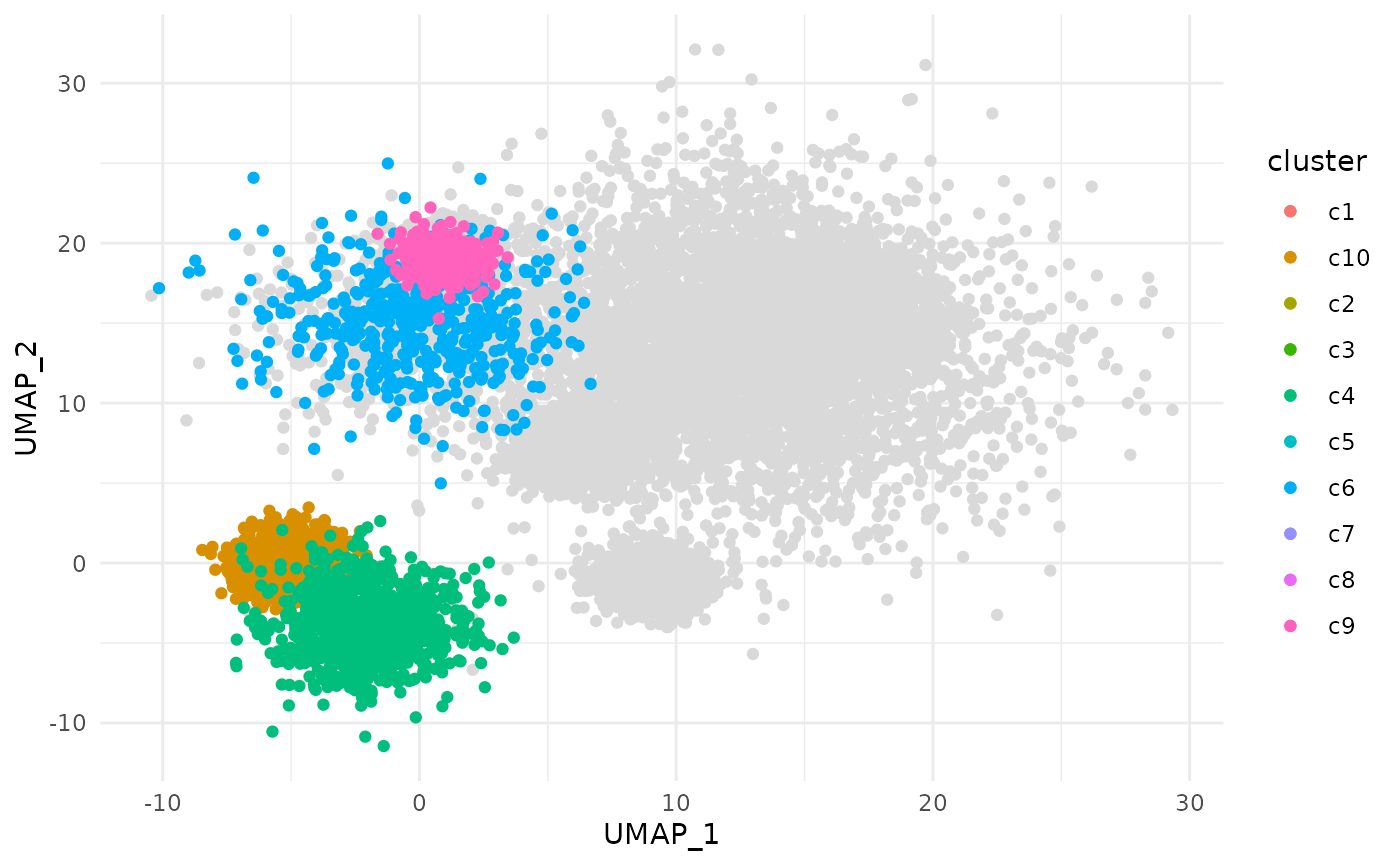## Basic Usage

geom_point_trace() is similar to ggplot2::geom_point(), but also includes the ability to outline points of interest. This is particularly useful when working with dense datasets that are prone to overplotting. This geom accepts normal ggplot2 graphical parameters with some modifications. fill controls the color of each point, color controls the outline color, and stroke controls outline width, similar to how filled shapes are modified for other ggplot2 geoms. Additional parameters including size, linetype, and alpha are also accepted.

library(ggplot2)
library(ggtrace)

p <- ggplot(
clusters,
aes(UMAP_1, UMAP_2, fill = cluster)
) +
theme_minimal()

p +
geom_point_trace(
color    = "black",
size     = 1,
linetype = 1,
alpha    = 1
)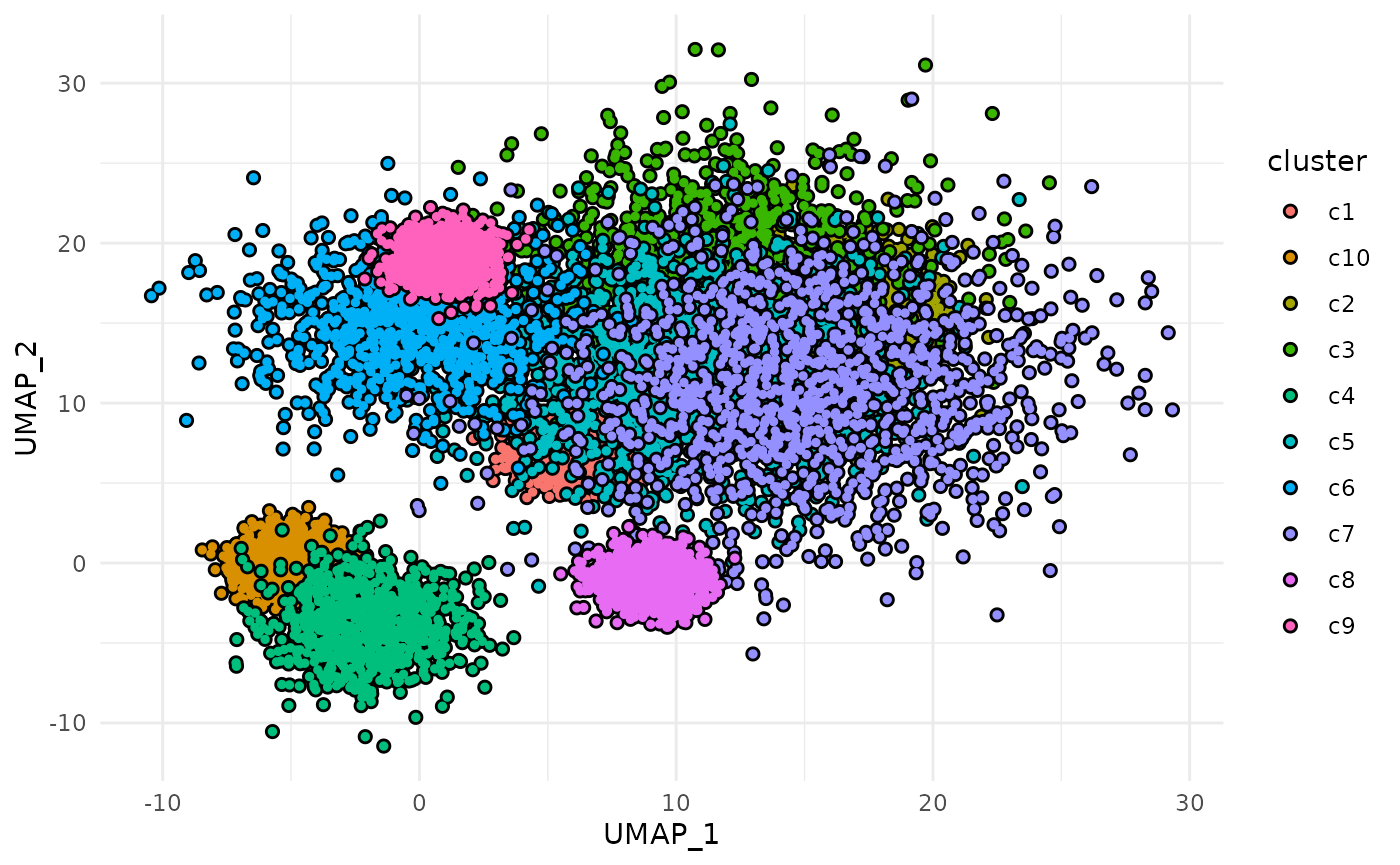## Aesthetics

Like other ggplot2 geoms, variables can be mapped to aesthetic attributes to modify the outline appearance.

ggplot(
clusters,
aes(UMAP_1, UMAP_2, color = cluster)
) +
geom_point_trace(
fill   = "black",
stroke = 2
) +
theme_minimal()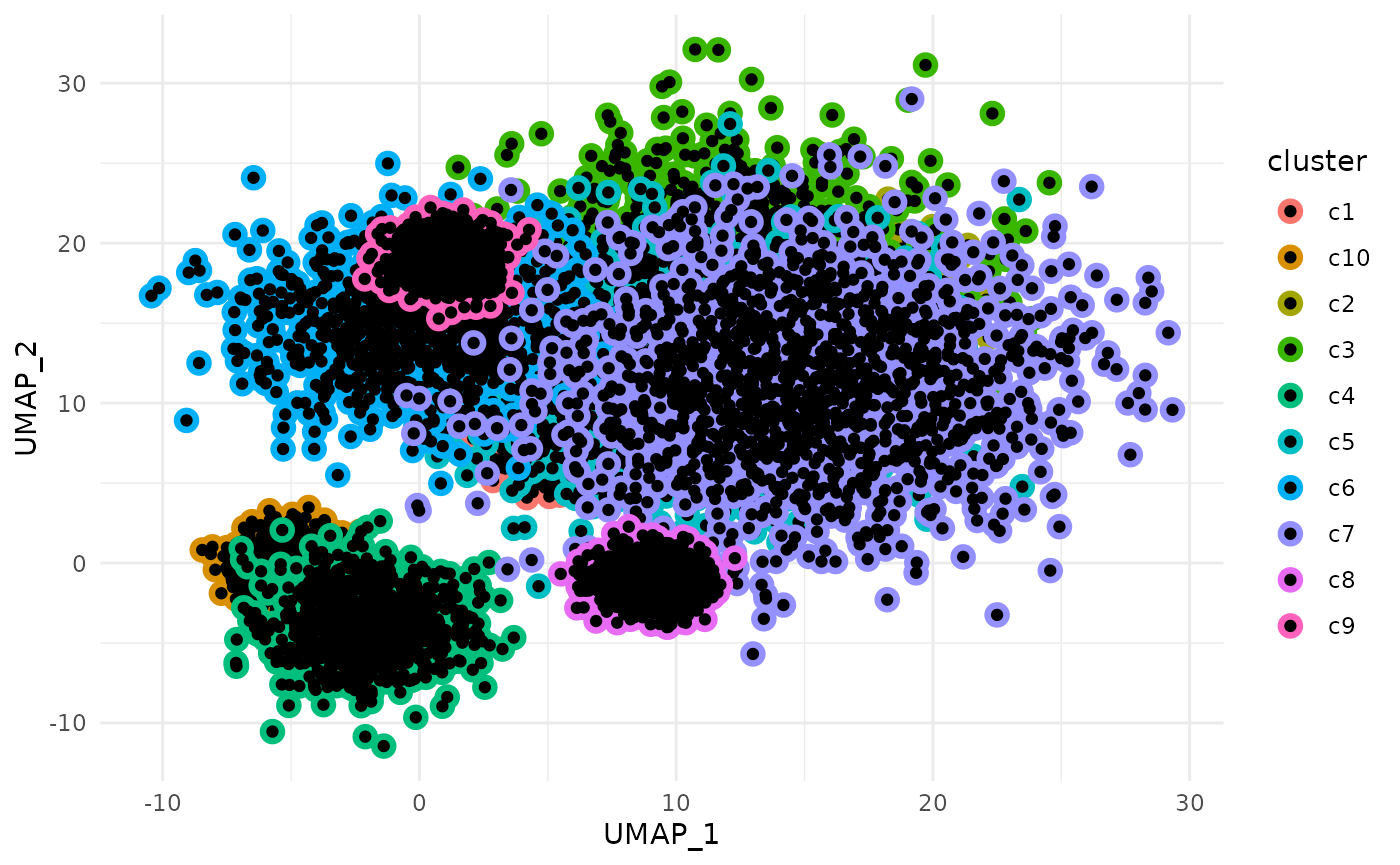By specifying group within aes(), outlines can also be added when coloring with a continuous variable. This is useful for visualizing points that are lightly colored.

p <- ggplot(
clusters,
aes(UMAP_1, UMAP_2, fill = signal, group = cluster)
) +
theme_minimal()

p +
geom_point_trace(stroke = 0.5) +
scale_fill_gradient(low = "white", high = "red")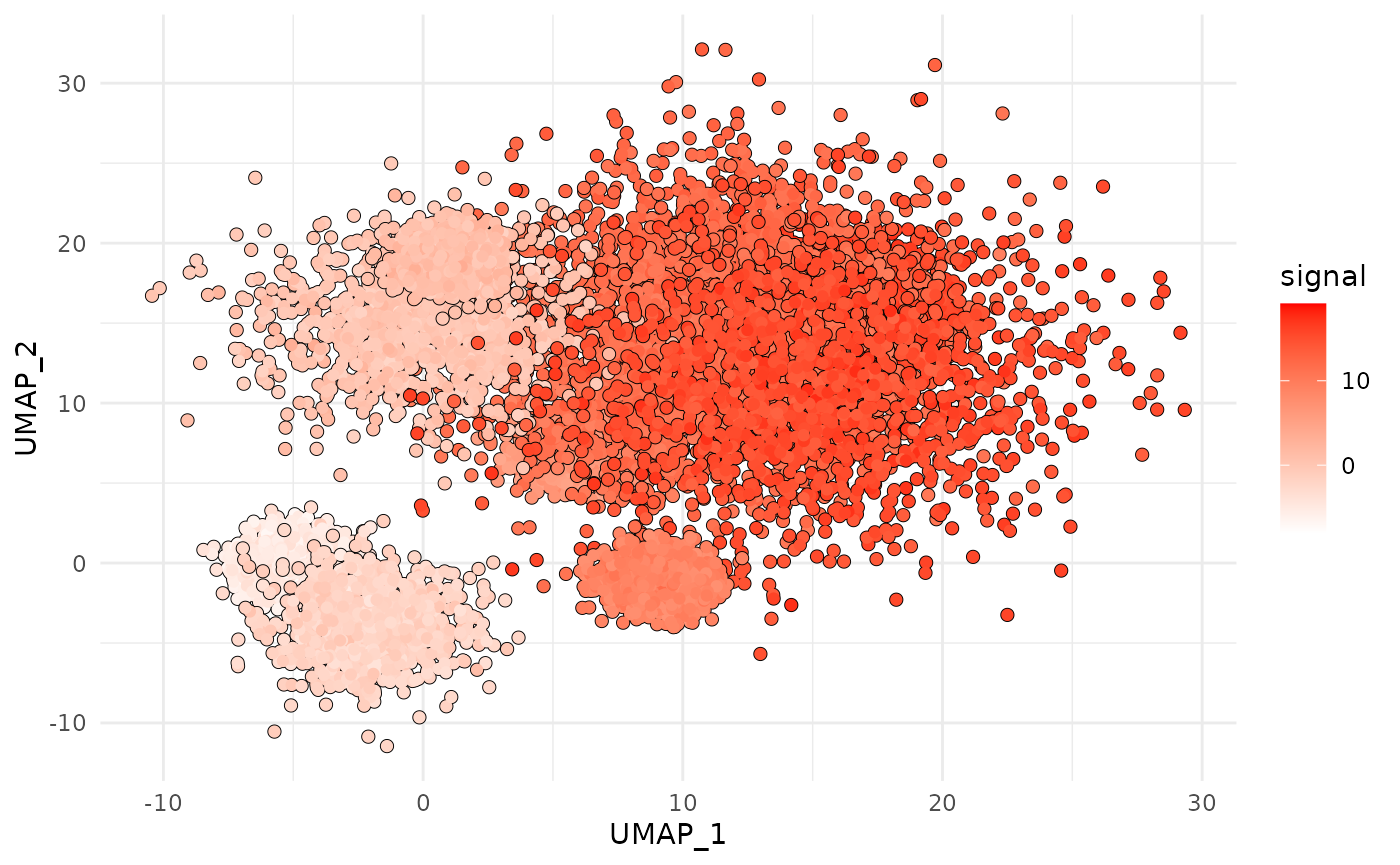Aesthetics can be further modified using the ggplot2 scale_*() functions.

p <- ggplot(
clusters,
aes(UMAP_1, UMAP_2, color = sample)
) +
theme_minimal()

p +
geom_point_trace(fill = "white") +
scale_color_manual(values = c("red", "#0072B2"))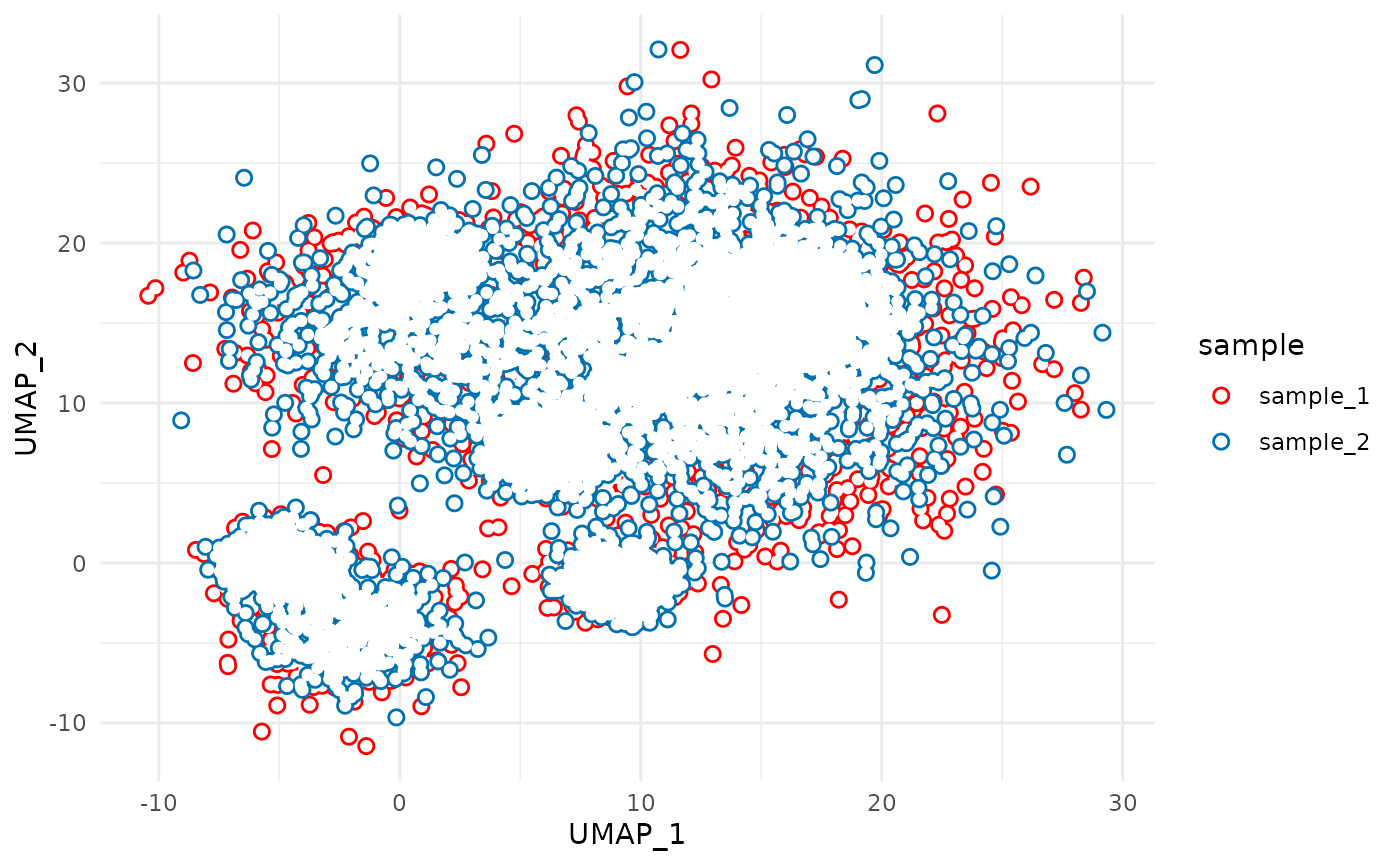## Position

The “position” of the outline can be modified with the trace_position argument. This can be “all”, “bottom”, or a predicate selecting the points to outline. By default all groups are outlined.

To only add a single outline around all points plotted, set trace_position to “bottom”.

p <- ggplot(
clusters,
aes(UMAP_1, UMAP_2, fill = cluster)
) +
theme_minimal()

p +
geom_point_trace(trace_position = "bottom")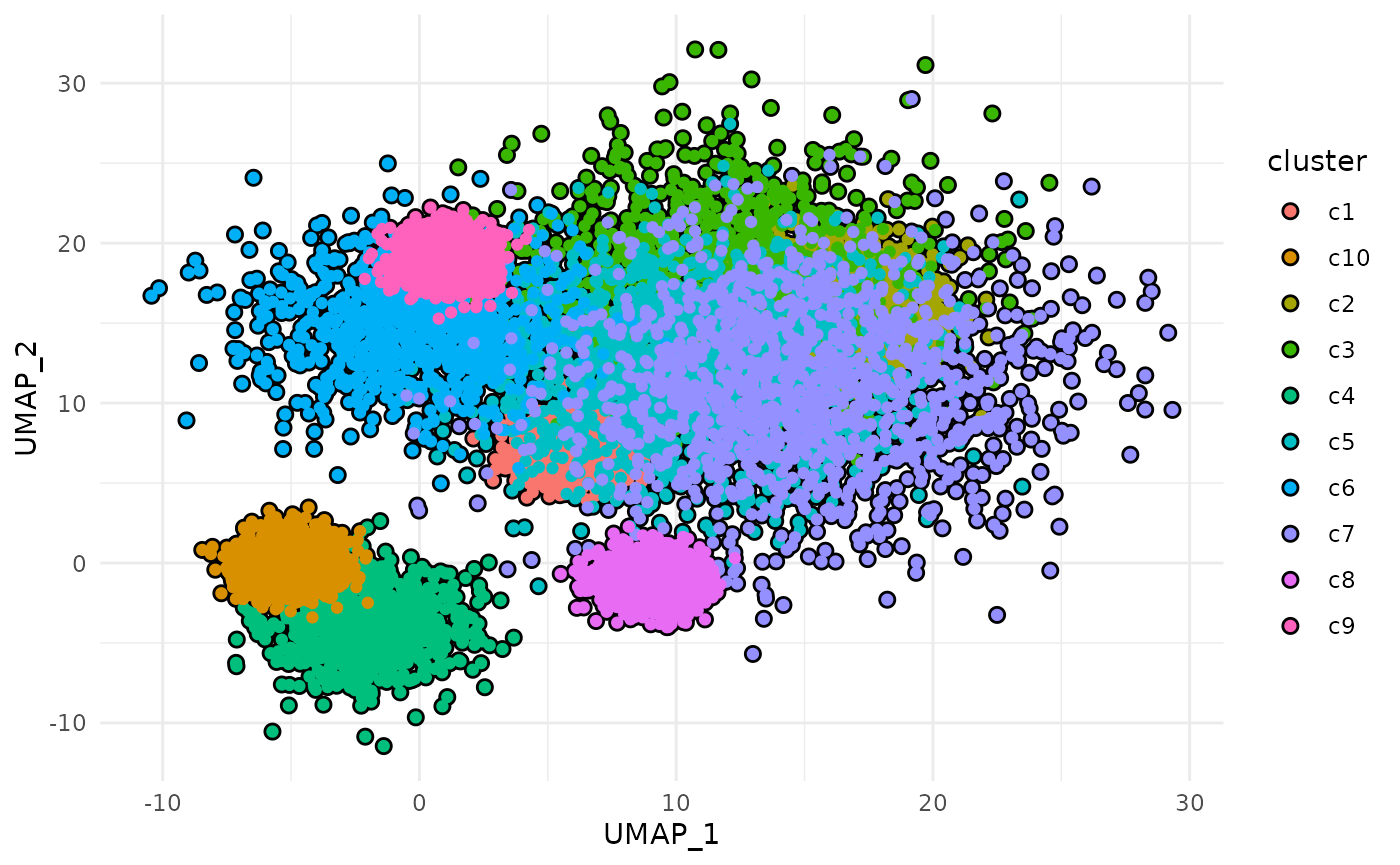A subset of data points can be highlighted by passing a predicate to trace_position. This must evaluate to TRUE or FALSE within the context of the input data.

p +
geom_point_trace(trace_position = signal < 0)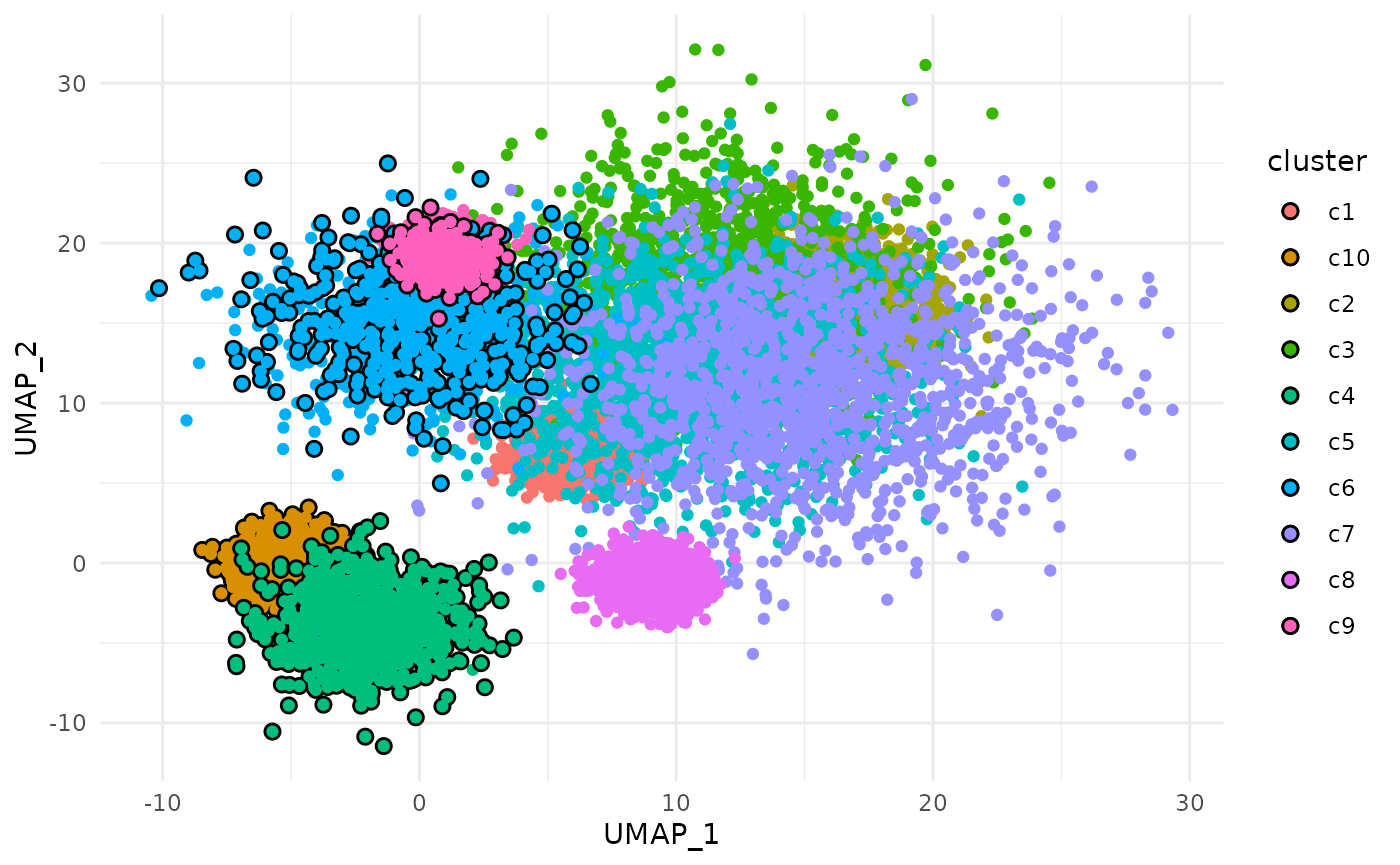The appearance of background points can be modified by passing a named list of parameters to background_params.

p +
geom_point_trace(
trace_position    = signal < 0,
background_params = list(color = NA, fill = "grey85")
)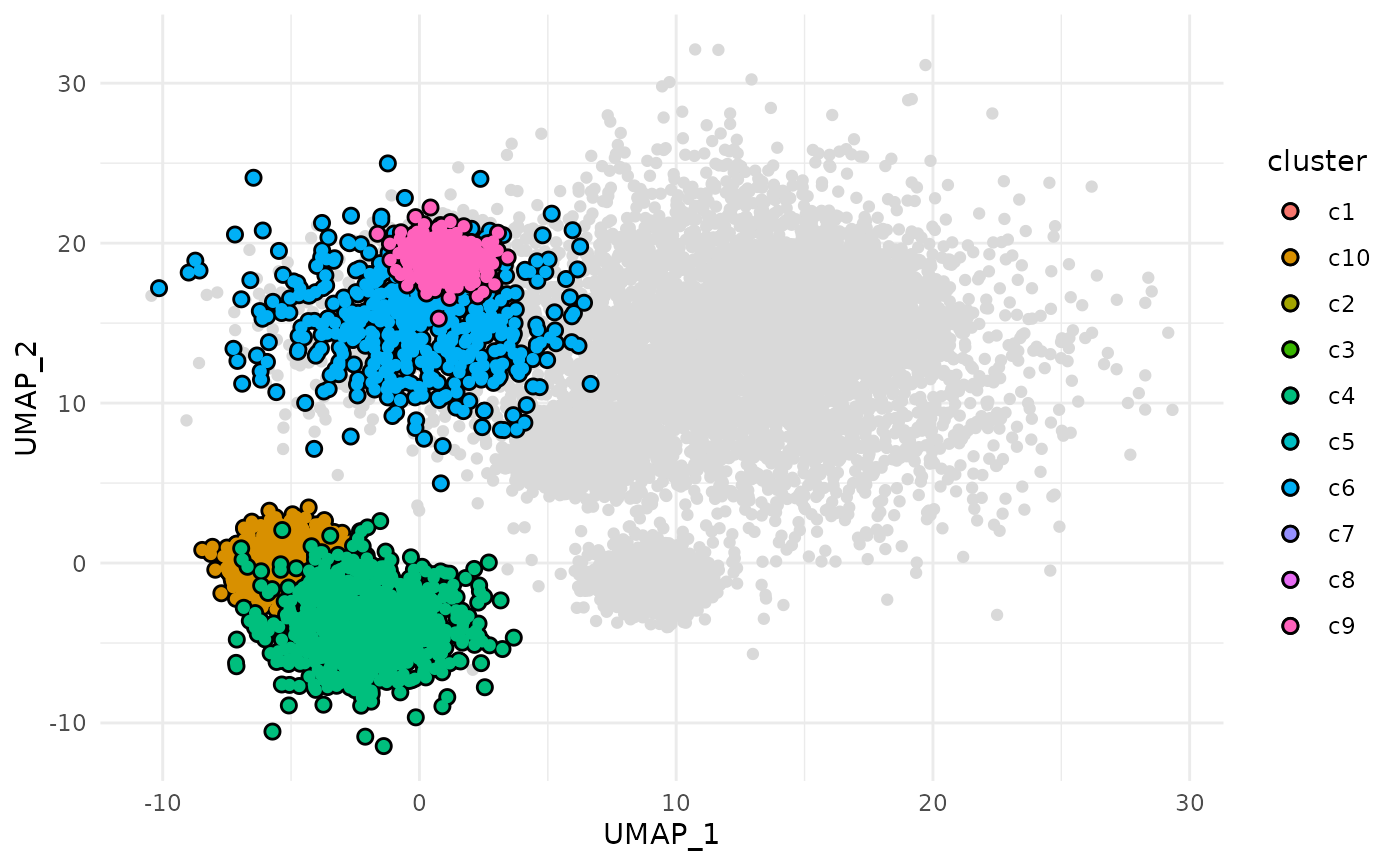Outlines can be removed by setting color to NA.

p +
geom_point_trace(
trace_position    = signal < 0,
color             = NA,
background_params = list(color = NA, fill = "grey85")
)